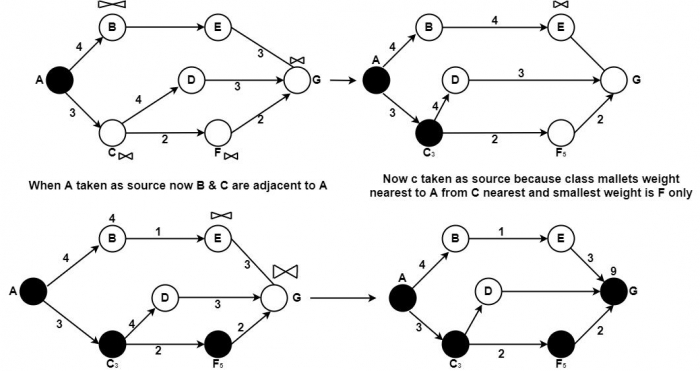# What is Dijikstra Algorithm?

The shortest path routing algorithm is an extensively set up non-adaptive routing algorithm that routes a packet dependent on Dijkstra's important shortest path algorithm. In this methodology, packets that appear at a node take one of the shortest paths to a specific destination on the system.

The shortcoming of this manner is that it does not consider the modification in the network traffic powerfully. For instance, queuing brings congestion in the middle nodes developing in packets being deferred before arriving at the destination. In this approach, it would be beneficial to advance packets to choose routes that cannot be the shortest path but can finally result in quicker transfer time.

The Shortest Path Routing (SPR) algorithm evaluates the routes depending on the cost metric represented in various matrices through the network to assess its routes.

Let V be a set of all the edges of the digraph and C[S, V] be the matrices of the least cost (of the shortest path) to any node to be evaluated. Initially, each edge (node), before adding its routes, sets the shortest path's cost to any other edge as infinity, i.e. . Suppose there are n edges in the digraph, each edge begins evaluating the cost  of the shortest paths to different nodes by selecting its neighbour x it is V – S with the lowest value of W (weight) from S to x. It evaluates the routes for each edge (S, x) which are neighbours of S, using the formula.

D [V] = min (D [V], D [V] + C(S, V)

C (S, V) are the costs of vertices connected to S. This procedure is extended until all the digraph edges are visited. When the algorithm is interrupted, all the nodes in the networks have to find each node's shortest path.

## Steps of Algorithm

• The source node is initialized and represented by a black circle.

• The neighbouring node's cost is determined and relabelled as a source node.

• It decided the smallest label by examining the adjacent node to make it permanent and take it as the source.

As shown in the example, we want to get G from A. So the steps are as follows −Now taken A as the first source and darken. Then C, then F and G is nearest to F and reaches the destination total weight is 7 from A to G and shortest path is (A, C, F, G) other than ABEG.